Equations
Trash Pandas
Order of Operations
Fractions & Decimals
Percent
Misc.
100

5x=-105

-21

100

Another name for a trash panda.

Racoon

100

Simplify the following expression: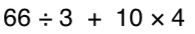100

-4 + -5

9100

Convert the following decimal to a fraction .38

17/50

100

What is 3/4 as a percent?

75%

100

greatest common factor of 12 and 30

6

200

p-54 =36

90

200

The color around a racoon's eyes.

Black

200

Simplify the following expression: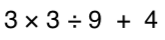200

14 + -12

2

https://www.khanacademy.org/math/arithmetic/arith-review-negative-numbers/arith-review-add-negatives-intro/v/adding-negative-numbers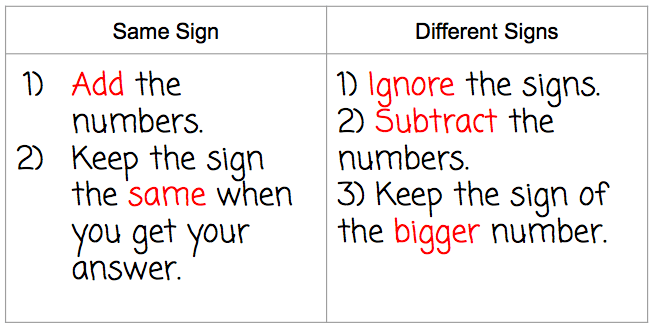200

Convert 4/10 into a decimal?

0.4

200

50% of 297 is what number?

150

200

least common multiple of 6 and 9

18

300

3x+1=-8

x=-3

300

Maximum speed of a racoon.

24km/h

300

3 + 42 * (3 + 2)

83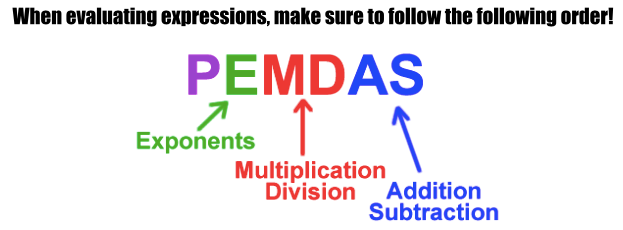300

-10 + 15

5300

Convert the following fraction to a decimal 7/20

.35

300

95% is what as a fraction in simplest form?

19/20

300

What is the greatest common factor of 64, 16, 32

16

400

6+ k/4 = 14

32

400

The flavor of a racoon resembles.

Dark meat from a turkey or chicken.

400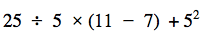26.25400

-18 + (-12) =

-30

400

3/4 + 1/6

11/12

400

What percent of 56 is 224?

25%

400

Find the Least common Multiple of 14, 21 and 7

42

500

27 =5x +2

x=5

500

Racoons do this before eating a meal.

Wash their food.

50012500

6 + (-2 + -5)

-1

500

Divide the following

2/5 divided by 7/10

4/7

500

12.5 is what percent of 1250?

1%

500

Find the Least Common Multiple of 3, 12, 6

12

Click to zoom